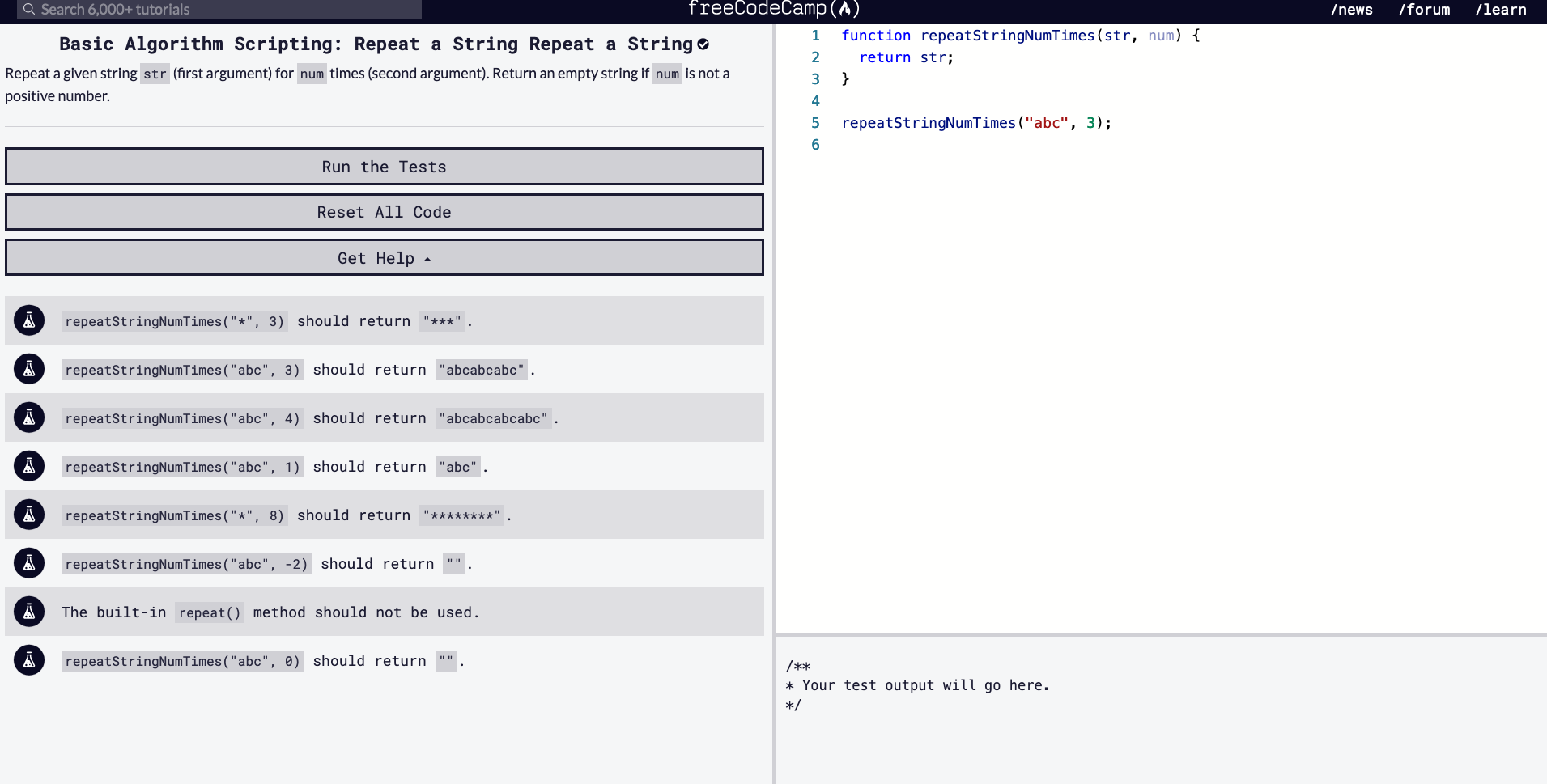## DEV Community

Viren B

Posted on • Updated on • Originally published at virenb.cc

# Solving "Repeat a String Repeat a String" / FreeCodeCamp Algorithm Challenges

Original post can also be found on my website, https://virenb.cc/fcc-007-repeat-a-stringLet's solve freeCodeCamp's Basic Algorithm Scripting Challenge, "Repeat a String Repeat a String"

## Our Starter Code (& Tests)

``````function repeatStringNumTimes(str, num) {
return str;
}

repeatStringNumTimes("abc", 3);
``````
``````// Tests
repeatStringNumTimes("*", 3) should return "***".
repeatStringNumTimes("abc", 3) should return "abcabcabc".
repeatStringNumTimes("abc", 4) should return "abcabcabcabc".
repeatStringNumTimes("abc", 1) should return "abc".
repeatStringNumTimes("*", 8) should return "********".
repeatStringNumTimes("abc", -2) should return "".
The built-in repeat() method should not be used.
repeatStringNumTimes("abc", 0) should return "".
``````

### Our Instructions

Repeat a given string `str` (first argument) for `num` times (second argument). Return an empty string if `num` is not a positive number.

## Thoughts

• We have two inputs, one being a `str` being a string, `num` being a number
• We have to return a string value
• Do not use `.repeat()`, a built-in method
• Need to check the value of `num`. If it is not a positive number, we must return an empty string

### Further Thoughts

There are definitely a few ways to solve this

Usually, I tend to try with a for loop first. Since one of the arguments is a number, we can run the for loop that amount of times

One of the instructions mentioned to return an empty string if `num` was not a positive number, so we can do a quick `if` statement to check and return an empty string

We can declare an empty string variable, create our for loop, run it `num` times, and add the `str` to the new empty string variable, `newStr`

Then ensure to return `newStr`

Let's look at that with some pseudo pseudocode

``````function repeatStringNumTimes(str, num) {
if num <= 0
return ""

create newStr variable, set to empty string
for loop: i = 0; i < num; i++
Add str value to newStr each loop

return newStr
}

repeatStringNumTimes("abc", 3);

``````

So the above code would run something like this:

``````repeatStringNumTimes("abc", 3);

if (num <= 0) return ""
// would return false and function would continue running since num is 3

let newStr = ""
// new empty string variable set

for (let i = 0; i < num; i++) {
newStr += str;
}
// Will loop 3 times since num is 3
// First loop - newStr == "abc"
// Second loop - newStr == "abcabc"
// Third loop - newStr == "abcabcabc"

return newStr; // "abcabcabc"
``````

## Solution

[SPOILER: SOLUTION TO CODE BELOW]

``````    function repeatStringNumTimes(str, num) {
let newStr = "";

if (num &lt;= 0) return "";

for (let i = 0; i &lt; num; i++) {
newStr += str;
}

return newStr;
}
``````

Repeat a String Repeat a String Challenge on FCC

FreeCodeCamp

Donate to FCC!

Solution on my GitHub